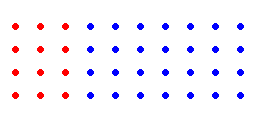# The Commutative, Associative and Distributive Laws (or Properties)

## The Commutative Laws (or the Commutative Properties)

The commutative laws state that the order in which you add or multiply two real numbers does not affect the result.

$a+b=b+a$

Example:

$\begin{array}{l}3+5=5+3=8\\ 20+\left(-3\right)=\left(-3\right)+20=17\end{array}$

The Commutative Law of Multiplication:

$ab=ba$

Example:

$\begin{array}{l}4\cdot 5=5\cdot 4=20\\ \left(-2\right)\left(8\right)=\left(8\right)\left(-2\right)=-16\end{array}$

## The Associative Laws (or the Associative Properties)

The associative laws state the when you add or multiply any three real numbers, the grouping (or association) of the numbers does not affect the result.

$\left(a+b\right)+c=a+\left(b+c\right)$

Example:

$\begin{array}{l}\left(2+3\right)+5=5+5=10\\ 2+\left(3+5\right)=2+8=10\end{array}$

The Associative Law of Multiplication:

$\left(ab\right)c=a\left(bc\right)$

Example:

$\begin{array}{l}\left(5\cdot 7\right)\cdot 6=35\cdot 6=210\\ 5\cdot \left(7\cdot 6\right)=5\cdot 42=210\end{array}$

## The Distributive Law

Let's see what happens if we do $4\left(7+3\right)$ :

$4\left(7+3\right)=4\left(10\right)=40$

This follows PEMDAS (the order of operations ).

But . . . $4\left(7\right)+4\left(3\right)=28+12=40$ , too, as seen in this picture:This is either a $4×10$ rectangle of dots, or a $4×3$ rectangle next to a $4×7$ .

Let's write this as

$4\left(7+3\right)=4\left(7\right)+4\left(3\right)$ .

We say we "distribute" the $4$ to the terms inside.

This translates to the general case:

$a\left(b+c\right)=ab+ac$ and $\left(b+c\right)a=ba+ca$

This is known as the Distributive Law or the Distributive Property . Click here for more examples of its use.